Jan Vrbik

## Simulating a Chain Sliding off a DesktopNBCDFPDF

### Equations of Motion

Consider a chain consisting of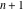point-like particles of the same mass (equal to 1, by a choice of units), connected bymassless, perfectly flexible, inelastic links of equal length (also equal to 1). The chain is laid on a table top, straight and perpendicular to the edge. Then the first particle is pulled (together with the rest of the chain) gently over the edge of the table. This causes the chain to start sliding down, due to gravity (also of unit magnitude), in a frictionless manner .

Let us assume now thatparticles have already left the table, and that their positions are defined byangles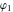,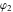, …,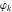by which the firstlinks deviate from the vertical, and by, the distance of the last particle to have left the table edge (is thus the angle of the hanging part of the corresponding link; the rest of it still lies flat on the table). Collectively, these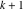variables are known as generalized coordinates , as they fully specify the position of every particle.

Now, using rectangular coordinates with the origin at the table’s edge, theaxis oriented vertically downward, and theaxis pointing horizontally, away from the table, we can compute the correspondingandcoordinates of each particle by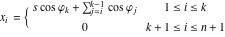(1)

and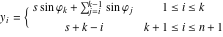(2)

The following program does exactly that.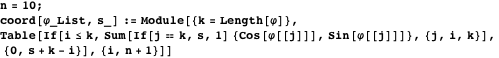The ensuing Lagrangian is the sum of the kinetic energies (that is,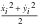, where a dot means a time derivative) of allparticles, minus the sum of their potential energies (which, individually, equal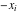), namely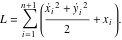(3)

This is easily converted to a Mathematica function.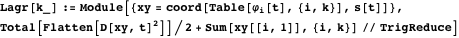The resulting equations of motion are obtained from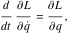(4)

whereis, one by one, each of the generalized coordinates. Here is the corresponding program.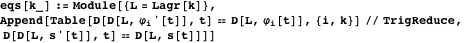For any particular set of initial values, namely a list ofangles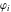(the first argument), their time derivatives (the second argument), distance(for our purpose set to 0 or, to avoid a technical problem, to a negligibly small value), and the derivative of(the third argument), we can solve these equations by the following program.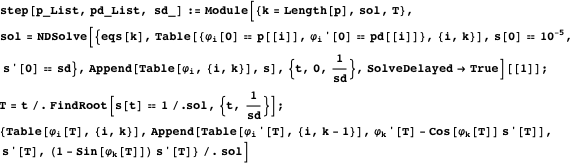The program advances the solution untilreaches the value of 1, that is, when the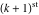particle has just left the desktop (technically, it is easier to let it go a bit further and then backtrack to time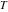defined by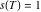). The program returns the new values of theangles, their derivatives, the horizontal velocity of theparticle (equal to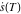; its vertical velocity is zero), and the rate of increase of the length of the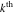link, given by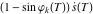. Note that the final value of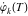has to be modified to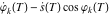, since as soon as theparticle leaves the table (even though, at this point, only by an infinitesimal amount),is no longer measured from the edge of the table; thelink now begins at this newly freed particle (this does not change the value ofitself, but it does change its derivative; the reader should be able to clearly visualize this). Furthermore, this also means that now theandparticles no longer keep their distance fixed (as the last item of the program’s output clearly indicates). Before we can continue with the next such step (to pull yet another particle off the desktop), we need to apply some new mechanism to quickly (preferably instantaneously) modify the velocities of these two particles, to make them maintain their fixed distance of 1 unit.

### Impulsive Solution

To achieve this, we replace the link connecting the last two “free” particles by a spring with a large spring constant of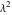and an equilibrium length of 1. Furthermore, we have to introduce two extra generalized coordinates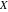and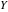(the rectangular coordinates of the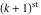point). This requires only the following minor modifications of the coord and Lagr routines (now combined into a single program).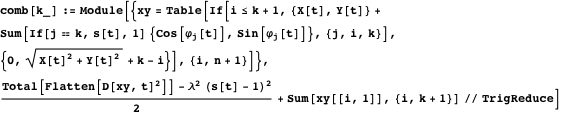The resulting Lagrangian is then easily converted into the corresponding equations of motion; unfortunately, the resulting solution exhibits undamped oscillations of(the length of thelink). To be able to continue with our simulation, we must first dampen these by adding to the corresponding differential equation a large frictional term of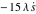(the coefficient of 15 was found empirically, to approximate critical damping). Adding friction will ultimately result in a small (and inevitable, for all models of this kind) loss of energy.

Then, we magnify the time scale by using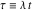as a new independent variable, and similarly replace each of the generalized coordinatesby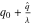, where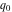is the corresponding initial value, andthus becomes the new dependent variable on a similarly magnified scale (its initial value is always 0). Finally, we let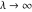and keep only-proportional terms of each equation (all other terms are, in this limit, discarded). The following program does all this, building the corresponding set of differential equations (yet to be solved).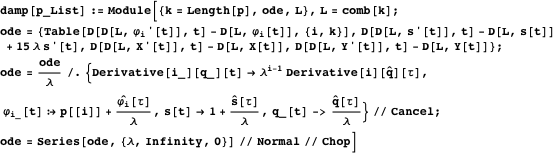All we need to do then is to integrate the resulting equations until a fully damped solution is attained (this means thatandhave reached the value of 0, and the other velocities no longer change). In real time, this happens instantaneously (a finite change ofrepresents only an infinitesimal increase in, due to thelimit); consequently, the values of our generalized coordinates remain fixed (again, a finite change indoes not modify the value of). This is no longer true for the corresponding derivatives—note that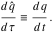(5)

The procedure thus changes, instantaneously, only the values of all generalized velocities. The actual solution is carried out by the program called impulse.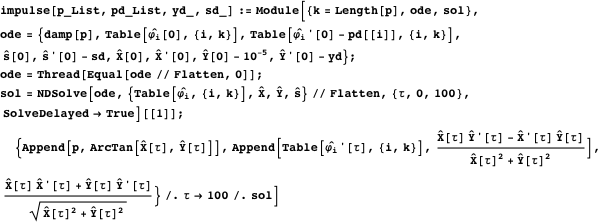The program takes the output of step as its arguments, initializes all variables to 0 (except for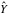, which needs to be made negligibly small) then, based on (5), initializes their derivatives (the program’s input), and advances the solution for 100 units of thescale (this appears sufficient to reach the desired equilibrium). If needed, the routine could be made more efficient by eliminating the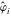as dependent variables (each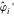can be expressed as a linear combination of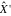,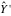, and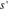).

### Simulation

Now we want to simulate a complete motion of such a chain until it loses touch with the table. The first step (until the second particle is about to lose contact with the desktop) is very simple; it results in the first particle being suspended vertically (i.e.,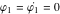) from the desktop’s edge, sliding down (and pulling the rest of the chain) at the speed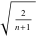(the chain’s kinetic energy must equal the loss of potential energy). After that, we have to alternate applying impulse and step to the current solution.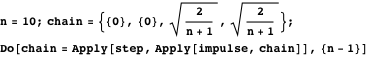The final shape of the chain (rotated by 90 degrees due to our coordinate system) is then displayed.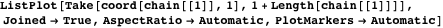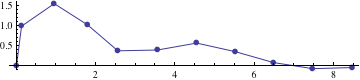To get a more realistic approximation to a real chain (or rope), one would have to substantially increase the value of. This would require rewriting our programs in a more efficient, task-dedicated manner. Nevertheless, even with the existing program, we can still produce (in several minutes) the following final profile of a 20-link chain.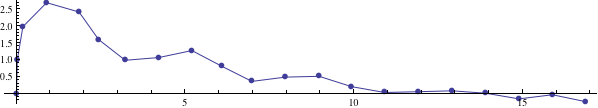One can prove that, in the limit as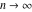, the chain will maintain an inverted L shape until exactly half of it has left the desktop, and only then start building the characteristic bulge of the final solution [3, 4]. This is to a good approximation true even with our 21 points, as we can see by displaying the chain’s shape after 10 particles have left the tabletop.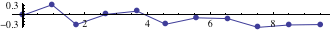We will leave it up to the reader to explore how (in)sensitive the solution is to the value of the damping constant, how much energy is lost in the impulse part of the solution, and other such interesting issues.

### References

  J. Vrbik, “Chain Sliding off a Table,” American Journal of Physics, 61(3), 1993 pp. 258-261. doi:10.1119/1.17442.  H. Lamb, Dynamics, 2nd ed., Cambridge: Cambridge University Press, 1961.  D. Prato and R. J. Gleiser, “Another Look at the Uniform Rope Sliding over the Edge of a Smooth Table,” American Journal of Physics, 50(6), 1982 pp. 536-539. doi:10.1119/1.12817.  J. R. Sanmartin and M. A. Vallejo, “Comment on ‘Another Look at the Uniform Rope Sliding over the Edge of a Smooth Table’,” American Journal of Physics, 51(7), 1983 p. 585. doi:10.1119/1.13170. J. Vrbik, “Simulating a Chain Sliding off a Desktop,” The Mathematica Journal, 2011. dx.doi.org/doi:10.3888/tmj.13-3.